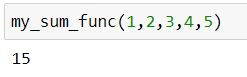Search
•Ekta Aggarwal

# Mastering args and kwargs in functions

Updated: Jan 25, 2021

While working with user defined functions in Python you will surely come across terminology like args and kwargs and why are they so important?

*args and *kwargs are the keywords using which you can create functions which can take variable length argument.

In this article we will learn about their utility and how they are implemented in Python in detail.

## Pre-requisites:

This tutorial assumes that you are already familiar about lists, dictionaries and creating user defined functions in Python. If you want to refresh your memory then you can refer to the following articles:

## args:

Let us understand with the help of an example:

Let me firstly create a UDF which takes 3 arguments x1, x2 and x3 and then returns in the square of the numbers:

```def my_func(x1, x2, x3):

def square_func(x):
"""Returns the square of a value."""
return x**2
return (square_func(x1), square_func(x2), square_func(x3))```
`print(my_func(1, 2, 3))`Now let us try to pass more arguments to the function:

`print(my_func(1, 2, 3,4,5))`

Python has thrown the TypeError: which means that function can take only 3 arguments while you are passing 5.But it is not feasible that number of arguments are always known to a programmer in advance. Thus to mitigate this Python has args . Let us create the same function, where we are passing *args as our function parameter.

```def my_func(*args):
squared_tuple = [i**2 for i in args]
return tuple(squared_tuple)

my_func(1,2,3,4,5)```

Alternatively, you can write the same function as:

```def my_func(*args):
squared_tuple = []
for i in args:
squared_tuple.append(i**2)
return tuple(squared_tuple)

my_func(1,2,3,4,5)```

*args parameter allows the programmer to pass non-keyworded arguments on which list operations can be carried out.### You can rename args to anything!

Let us define another sum function with args.

```def my_sum_func(*args):
summed = sum(args)
return summed

my_sum_func(1,2,3,4,5)```Let us create another function where instead of *args I have written *my_vector as the argument. But the resultant output is same. Thus you can use any other name as well. args is just a random name.

Do remember! You have to put a * before the name to tell Python that UDF will have parameters of varying length.

```def my_sum_func(*my_vector):
summed = sum(my_vector)
return summed

my_sum_func(1,2,3,4,5)```## kwargs:

kwargs is the short form of keyword arguments. It is denoted by ** . It has a similar utility to args but it is not used for lists, rather it is used for working with dictionaries.

When number of items in our dictionary is not defined then we can use **kwargs.

Let us take an example to understand how a UDF with kwargs looks like:

```def my_func(**kwargs):
"""Printing Items in kwargs"""
print(kwargs.items())
"""Printing Keys in kwargs"""
print(kwargs.keys())
"""Printing Values in kwargs"""
print(kwargs.values())

for k,v in kwargs.items():
print(k," - ",v )```

Our above UDF will take a dictionary of varying number of keyworded arguments (i.e. items) and would print the items, keys, values and concatenate key and values.

Let us pass a dictionary with 2 items to our above UDF.

`my_func(country = "U.S.A", capital = "Washington D.C.")`### You can rename kwargs too!

Like args, we can also rename kwargs. I have recreated the above function using **some_random_name instead of **kwargs which will result in same output as above.

```def my_func(**some_random_name):
"""Printing Items in kwargs"""
print(some_random_name.items())
"""Printing Keys in kwargs"""
print(some_random_name.keys())
"""Printing Values in kwargs"""
print(some_random_name.values())

for k,v in some_random_name.items():
print(k," - ",v )```

### Order parameters in our UDF

In a UDF you need to keep in mind the following order:

• Normal arguments

• *args

• **kwargs

Our appropriate UDF would be :

```def my_func(a=10,*args,**kwargs):
print('Value of a is: ', a)
print('Value of args is: ', args)
print('Value of kwargs is: ' , kwargs)

my_func(200,50,30,24,lucky_number = 7)```Switching the order would lead to errors:

```def my_func2(a=10,**kwargs,*args):
print('Value of a is: ', a)
print('Value of args is: ', args)
print('Value of kwargs is: ' , kwargs)```### Unpacking args and kwargs

Let us create a function and two lists:

```def my_func(*args):
print(args)
my_list = [100,200,300,400];
my_second_list = [10000,20000,30000]```

Let us try calling the function:

`my_func(my_list, my_second_list)`

Notice that in the output we only have two objects in args where both the objects are lists.In order to pass the elements of the list as individual elements we need to unpack the list.

A list is unpacked by writing a * before its name.

A dictionary is unpacked by writing a ** before its name.

`my_func(*my_list,*my_second_list)`Tags:

See All